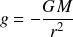# Gravitational acceleration

Gravitational acceleration characterizes the strength of a gravitational field. The formula quantifies the gravitational field in terms of its influence on the acceleration of a second body.

Gravitational acceleration potential is determined by the mass density and distance operators acting on the maximum force potential, giving the elementary form of the equation

## Historical formula

The elementary formula can be derived from the historical gravitational acceleration formula which uses the gravitational constant. The historical form of the equationcan be re-stated in elementary form by replacing the gravitational constant with natural units of length, mass, and time. The elementary formulacan then be grouped into operators and potentialsThe following illustration shows how the historical formula transforms into the natural formula with operators and potential.

Gravitational acceleration: conversion from historical to natural formula

Gravitational acceleration